we can compute the radial wave functions Here is a list of the first several radial wave functions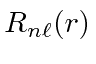.For a given principle quantum number,the largestradial wavefunction is given byThe radial wavefunctions should be normalized as below.* Example: Compute the expected values of,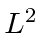,, andin the Hydrogen state.*

The pictures below depict the probability distributions in space for the Hydrogen wavefunctions.The graphs below show the radial wave functions. Again, for a giventhe maximumstate has no radial excitation, and hence no nodes in the radial wavefunction. Asgets smaller for a fixed, we see more radial excitation.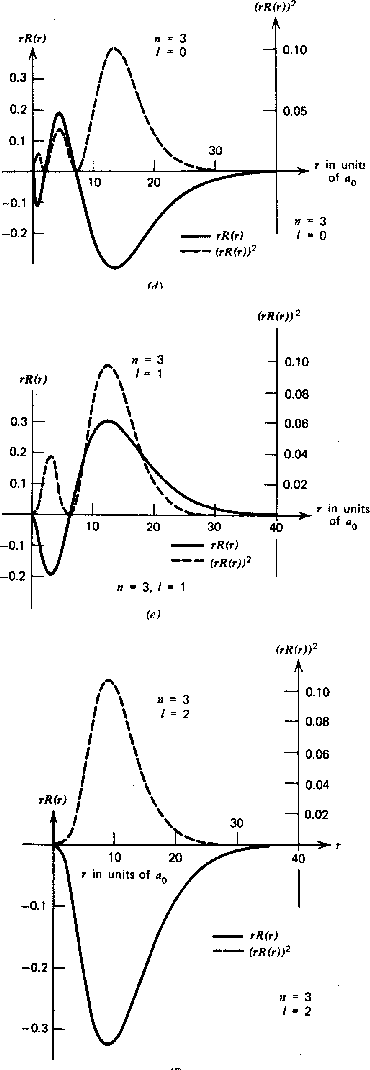A useful integral for Hydrogen atom calculations is.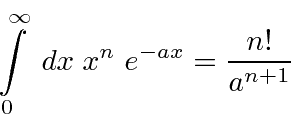* Example: What is the expectation value ofin the state?*

* Example: What is the expectation value of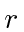in the state?*

* Example: What is the expectation value of the radial component of velocity in the state?*

Jim Branson 2013-04-22